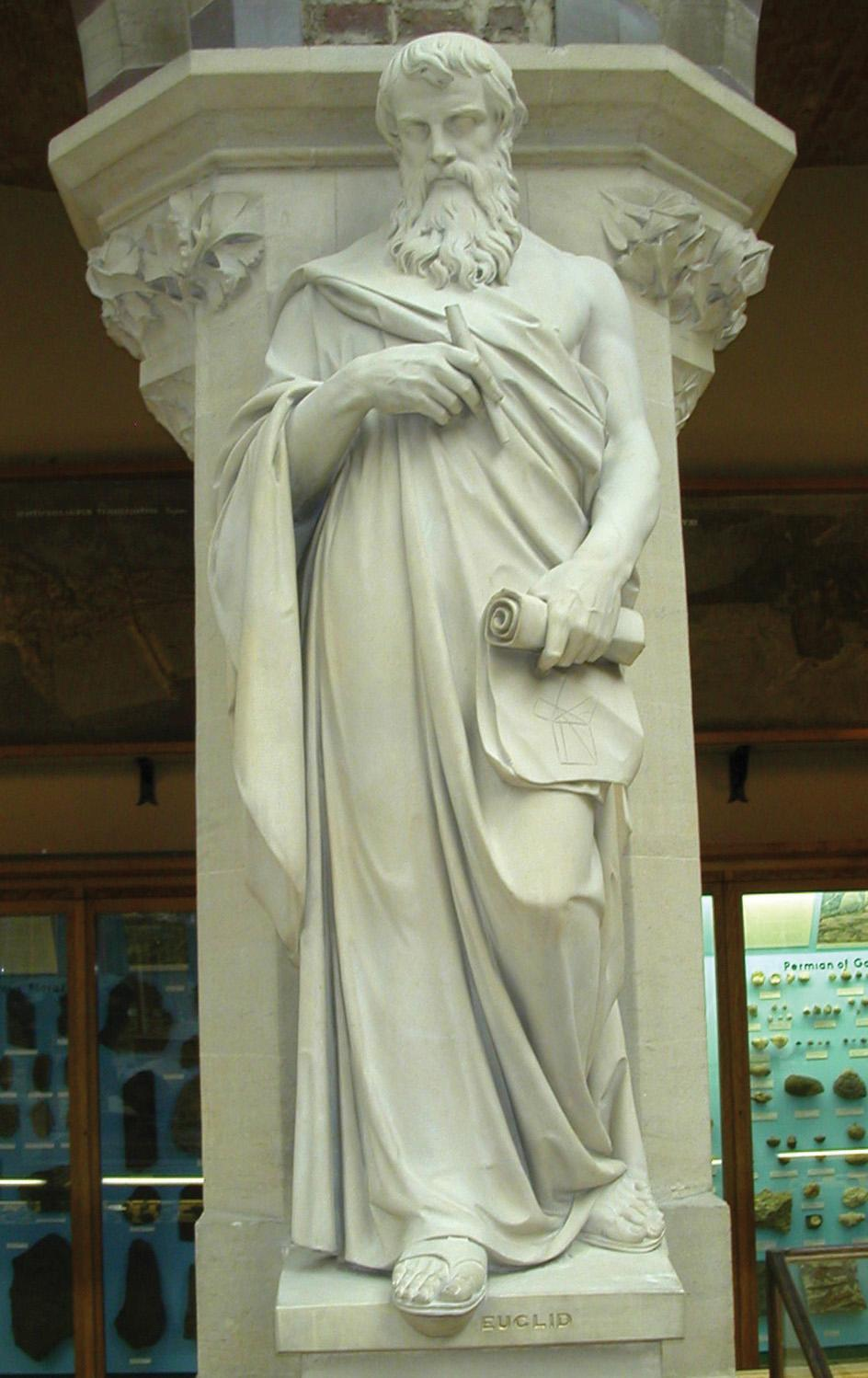The square root of a number N is a number which, when squared (multiplied by itself), results in N. How did the ancient Greeks find square roots? Their method is nowadays called a ‘Greek ladder’, which uses whole numbers throughout.A 19th-century statue of the ancient Greek mathematician Euclid of Alexandria. Photo: Wikipedia

To illustrate Greek ladders, let us find the square root of five, so that N is five. We first find m, the largest number that, when squared, results in a number less than N.

In our case, m is two, because two times two is four, which is less than five, but three times three is nine, which is larger than five. Then we can find q, the difference between N and the square of m. In this case, q is five minus the square of two, which is one.

A sequence of numbers is then produced according to the following two rules:

1) The first two numbers in the sequence are zero and one.

2) Any other number in the sequence is the previous number times twice m, plus the number before that times q.

For the square root of five, remembering that m is two and q is one, we obtain the sequence:  0, 1, 4, 17, 72, 305, 1292, 5473, … for example, the number 305 in the above sequence is 72, the previous number in the sequence, times twice m (four), plus 17 (the number before 72 in the sequence) times q (one).

The Greeks stopped this sequence when the numbers became prohibitively large for them to be worked out efficiently. Once the sequence is stopped, the square root of five is obtained by dividing the last two numbers in the sequence by each other, then subtracting m. This means that the square root of five is, roughly, 5473 divided by 1292, minus two, which is the fraction 2889 / 1292, which, in today’s notation, is 2.236068111….

Comparing this number with the decimal expansion of the square root of five, which is 2.236067977…, we notice that 2889 / 1292 is the square root of five correct to six decimal places! Moreover, to obtain an even better approximation, we simply go further in the above sequence of whole numbers.

It is not known how the Greeks discovered this method. Since the above sequence is infinitely long, they might have concluded that there cannot exist an exact fraction for such square roots, possibly leading them to discover irrational numbers.

Nowadays, we have more sophisticated methods to approximate numbers such as square roots at our disposal. Scientists are actively pursuing further improvement in efficiency of these methods.

Dr Alexander Farrugia is the mathematics subject coordinator at the University of Malta Junior College.

## Did you know?

• The mathematics introduced to the world by ancient Greece is fundamental to today’s knowledge of geometry. The idea of formal proof in mathematics also stemmed from ancient Greece.

• Perhaps the most important work that ancient Greece gifted to the modern world is Euclid’s Elements. Written in 300BC, it is a collection of 13 books containing most, if not the entire knowledge, of the mathematics that was known to the ancient Greeks at that time. It is considered as the first mathematics book presenting its contents via definitions, from which theorems are deduced using logical arguments.

• Another champion of ancient Greek mathematics is Archimedes of Syracuse (250BC). Among other achievements, Archimedes contributed a proof that pi is a number between the fractions 223/71 and 22/7, the latter of which is still used as an approximation of pi to this day.

For more trivia, see: www.um.edu.mt/think

## Sound bites

• Diophantus of Alexandria, a mathematician from ancient Greece, was the author of several books called Arithmetica, the majority of which are lost. In the paper ‘J. Christianidis & A. Megremia. Tracing the Early History of Algebra: Testimonies on Diophantus in the Greek-speaking World (4th-7th century CE), Historia Mathematica, 47 (2019), 16-38’, the authors challenge the common belief that Diophantus’s Arithmetica is an isolated phenomenon in the history of ancient Greek mathematics. This is done by investigating testimonies that suggest that Diophantus’s heritage was already present in the intellectual setting of the Greek-speaking world during the late antique times.

• Conic sections constitute a major contribution to mathematics by the ancient Greeks. The paper ‘E. Rinner. Ancient Greek Sundials and the Theory of Conic Sections Reconsidered. In: John Steele, Mathieu Ossendrijver (eds.), Studies on the Ancient Exact Sciences in Honor of Lis Brack-Bernsen, Berlin Studies of the Ancient World, 44 (2018), 165-182’, argues that craftsmen who built sundials in the Roman period had basic knowledge of conic sections.